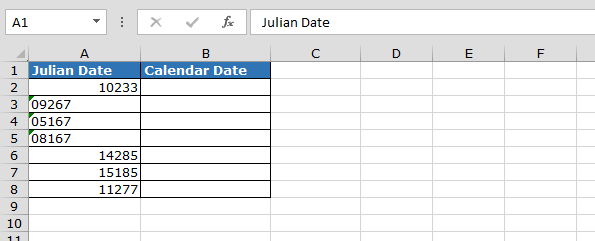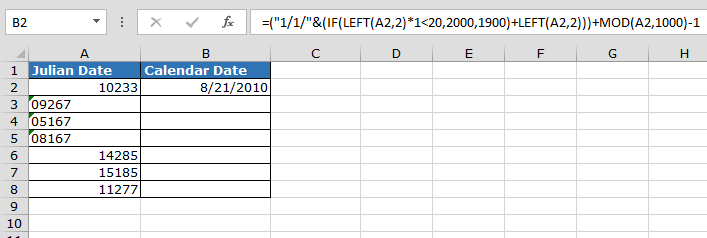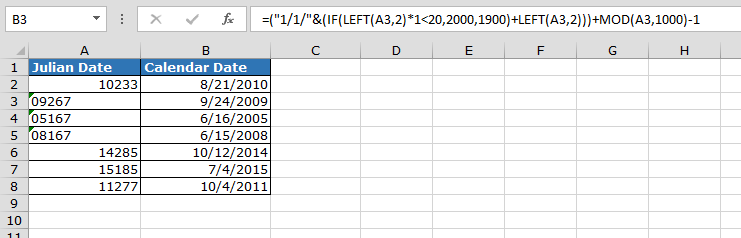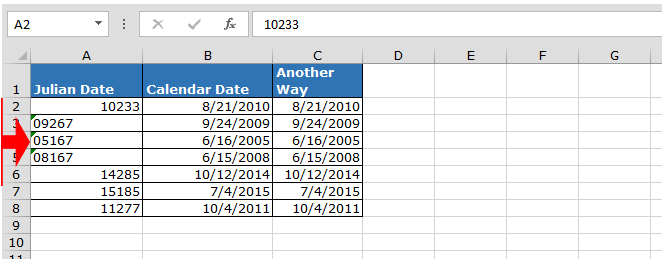# Convert Julian Date to a Calendar Date in Microsoft Excel

In this article you’ll learn, how to convert a Julian date to a calendar date.

What is Julian date?

Julian date calendar was introduced by Julius Caesar. The length of Julian date is 5, in which first 2 digits represt the year and last 3 digits represent the day of the year.

For example:-

• 1st January 2008, Julian date 08001. Where “08” being the year and “001” being the date

To convert the Julian date format to a calendar date, we use IF, LEFT and MOD function in Microsoft Excel.

Let’s take an example,

In Column A we have Julian date and in column B we want to convert Julian date into calendar date.• Enter the formula in cell B2
• =("1/1/"&(IF(LEFT(A2,2)*1<20,2000,1900)+LEFT(A2,2)))+MOD(A2,1000)-1
• Press Enter
• The function will convert the Julian date format in to Calendar date•  Copy the same formula by pressing the key Ctrl+C and paste in the range B3:B10 by pressing the key Ctrl+V on your keyboardFormula Explanation:-

• (IF(LEFT(A2,2)*1<20,2000,1900)+LEFT(A2,2))) :- This part of formula will help to get the year
• MOD(A2,1000) :- This part of formula will help to get the number of days
• By using rest part formula will calculate the date as number, because excel has stored the date as numbers.

In this way, we can convert the Julian date to a calendar date in Microsoft Excel.

Important Note:- To convert the 4 digit’s Julian date into calendar date we have to convert Julian date into text format.

Take a look at the below example,We would love to hear from you, do let us know how we can improve, complement or innovate our work and make it better for you. Write us at info@exceltip.com

1.